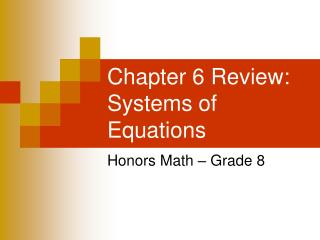Download PresentationChapter 6 Review: Systems of Equations

# Chapter 6 Review: Systems of Equations - PowerPoint PPT Presentation

Chapter 6 Review: Systems of Equations. Honors Math – Grade 8. Important Vocabulary Systems of equations Elimination using Addition/Subtraction Intersecting and Parallel Lines Substitution.I am the owner, or an agent authorized to act on behalf of the owner, of the copyrighted work described.
Download Presentation## Chapter 6 Review: Systems of Equations

Download Policy: Content on the Website is provided to you AS IS for your information and personal use and may not be sold / licensed / shared on other websites without getting consent from its author.While downloading, if for some reason you are not able to download a presentation, the publisher may have deleted the file from their server.

- - - - - - - - - - - - - - - - - - - - - - - - - - E N D - - - - - - - - - - - - - - - - - - - - - - - - - -
Presentation Transcript
1. Chapter 6 Review: Systems of Equations Honors Math – Grade 8

2. Important Vocabulary Systems of equations Elimination using Addition/Subtraction Intersecting and Parallel Lines Substitution

3. Use the graph to determine whether each system is consistent or inconsistentand if it is independent or dependent.

4. The following table states the best time to use a method for solving a system of equations.

5. Choose the best method and solve each system of equations.

6. Choose the best method and solve each system of equations.

7. Choose the best method and solve each system of equations.

8. Distribute At a sale, Sarah bought 4 T-shirts and 3 pairs of jeans for \$181. Jenna bought 1 T-shirt and 2 pairs of jeans for \$94. The T-shirts were all the same price and the jeans were all the same price. How much did each item cost? Define the Variables Let t = the cost of a t-shirt and j = the cost of jeans.. Write a system of equations. 4 t-shirts + 3 jeans = 181 4t + 3j = 181 1 t-shirt + 2 jeans = 94. 1t + 2j = 94 1. Write the equations in column form. Multiply to eliminate a variable. The t variable is eliminated because 4 - 4 = 0 - 4( ) Solve the equation 2. Since the coefficients of t are 4 & 4 (the same), subtract the equations. j = 39 3. Now substitute j = 39 in either equation and solve. A t-shirt costs \$16 and a pair of jeans cost \$39.

9. In 2000, the number of film cameras sold was 20 million and the number of digital cameras sold was 4.7 million. Since then, the number of film cameras has decreased at an average rate of 2.5 million per year and digital cameras has increased at an average rate of 2.6 million per year. In how many years will the sale of digital cameras equal the sale of film cameras? Define the Variables Let y = the number of cameras sold and x = the number of years after 2000. Write a system of equations. Film decreased 2.5 per yr. y = 20 – 2.5x Digital increased 2.6 per yr y = 4.7 + 2.6x • Write the equations in column form. You may • eliminate if equations are not in standard form 2. Since the coefficients of yare 1 & 1 (the same), subtract the equations. The y variable is eliminated because 1 - 1 = 0 - Solve the equation x = 3 In 3 years (or 2003), the number of digital cameras sold will equal the number of film cameras sold.

10. For the Future Teachers of America fund-raiser, Denzel sold subs for \$3 and pizzas for \$5. He sold 11 more subs than pizzas and earned a total of \$233. How many pizzas and subs did he sell? Earned a total of \$233. 3s + 5p = 233 He sold 11 more subs than pizzas. s = p + 11 Define the Variables Let s = the number of subs sold & p = the number of pizzas sold. Write a system of equations. One equation is solved for s; Substitute s= p+11 Substitute p + 11 for s in the first equation. 2. Now substitute p = 25 in either equation and solve. Distribute and group like terms. Solve. p = 25 Denzel sold 25 pizzas and 36 subs.

11. Mara recycled 9 pounds of aluminum cans and 26 pounds of newspapers and earned a total of \$3.77. Ling recycled 9 pounds of aluminum cans and 114 pounds of newspaper and earned a total of \$4.65. What was the price per pound of each? Define the Variables Let a = the cost of aluminum n = the cost of newspaper. Write a system of equations. 9 lbs aluminum & 26 lbs news = 3.77 9a + 26n = 3.77 9 lbs aluminum & 114 lbs news=4.65 9a + 114n = 4.65 1. Write the equations in column form. 2. Since the coefficients of a are 9 & 9 (the same), subtract the equations. - The a variable is eliminated because 9 – 9 = 0 Solve the equation n = .01 3. Now substitute n = .01 in either equation and solve. Aluminum cans cost \$0.01 per pound and newspapers cost \$0.39 per pound